Arithmetic Progression

Chapter 8 Class 11 Sequences and Series
Serial order wise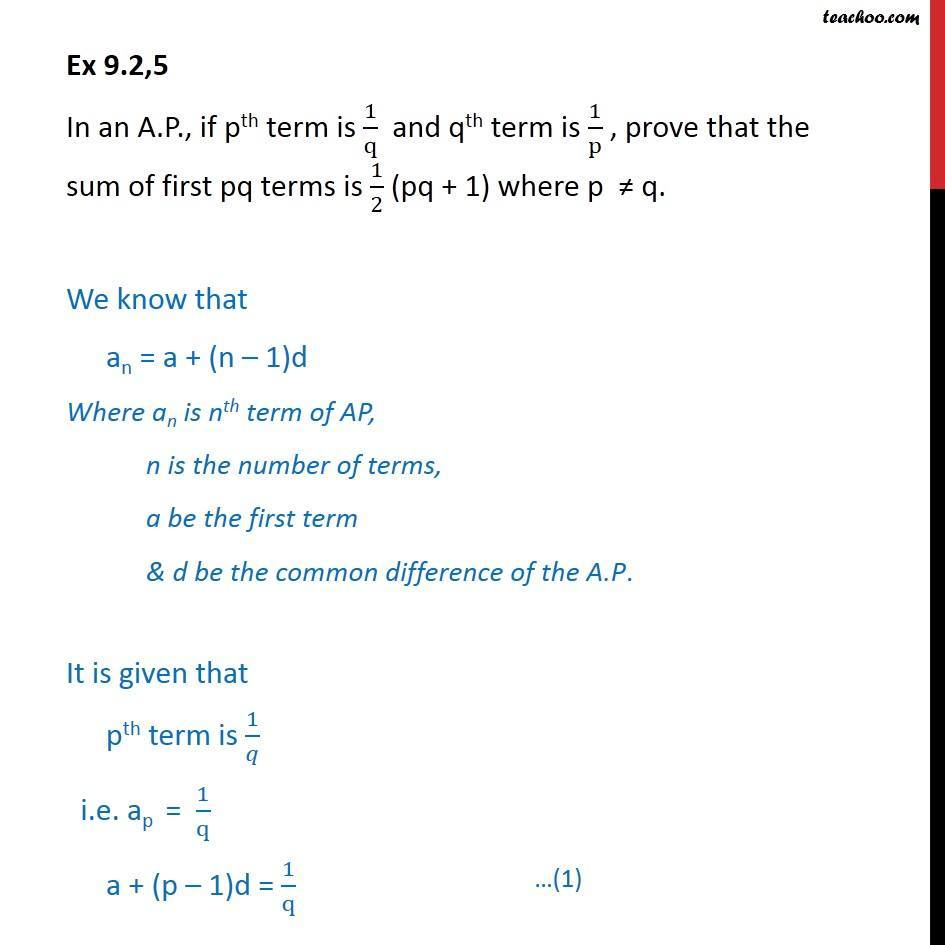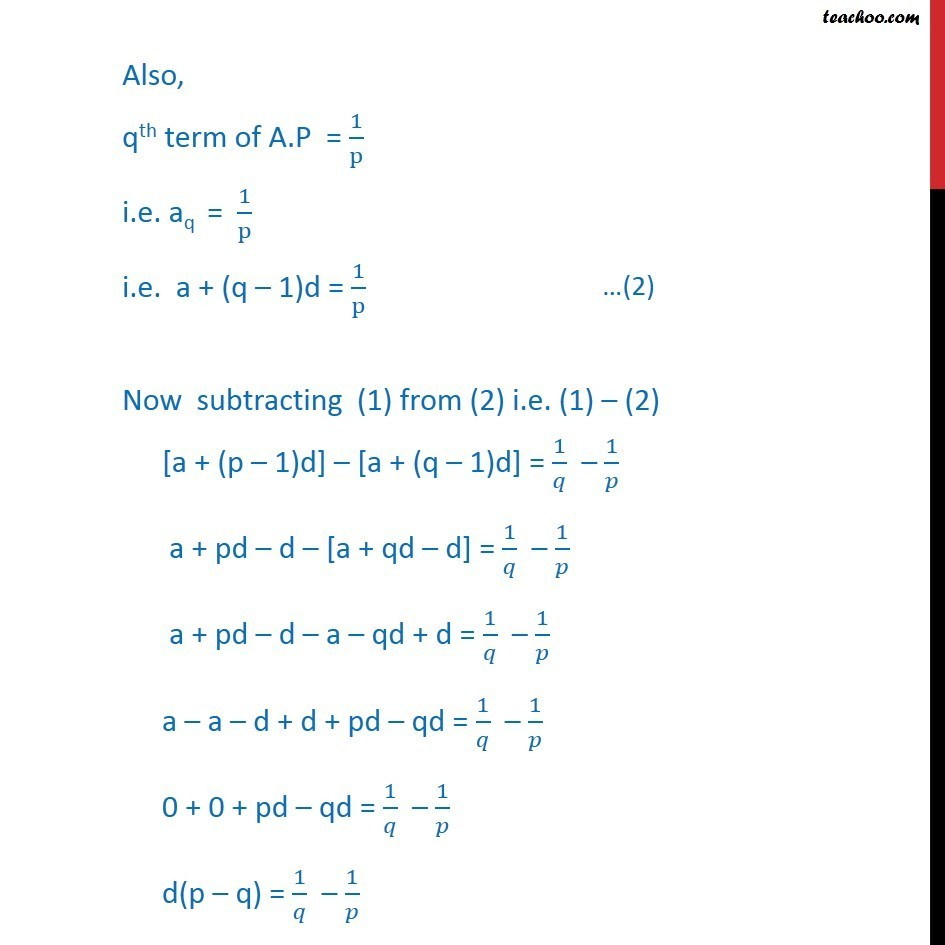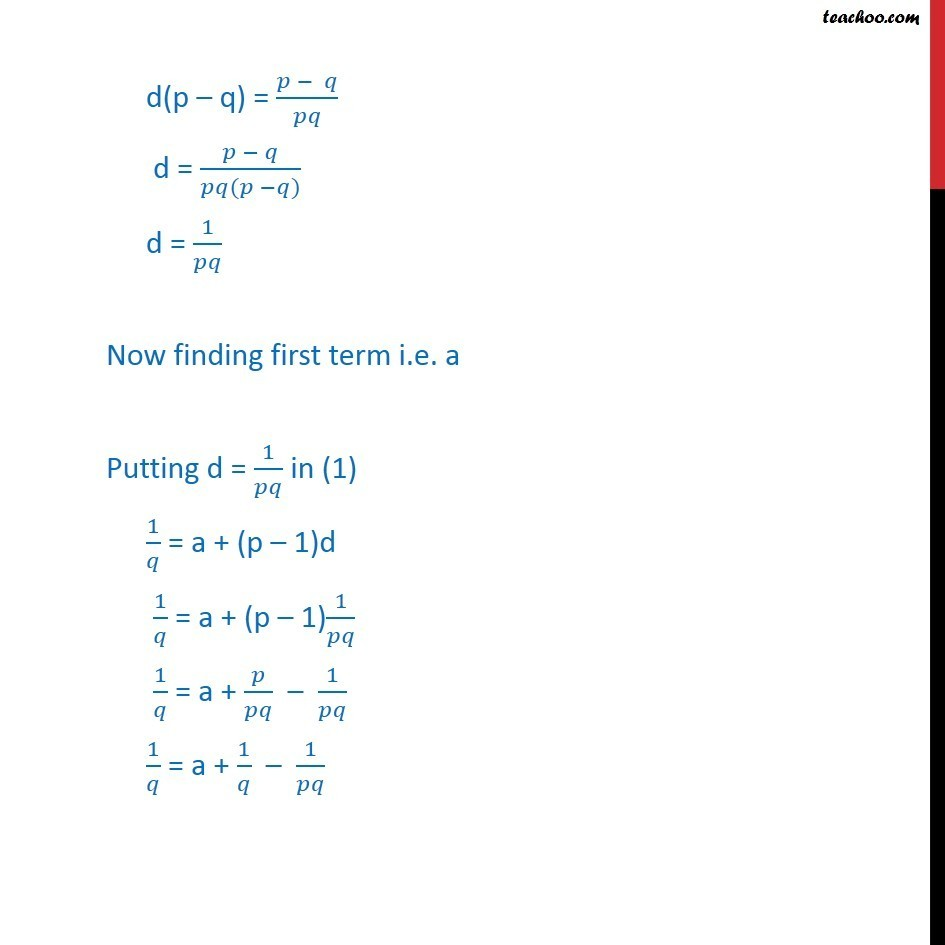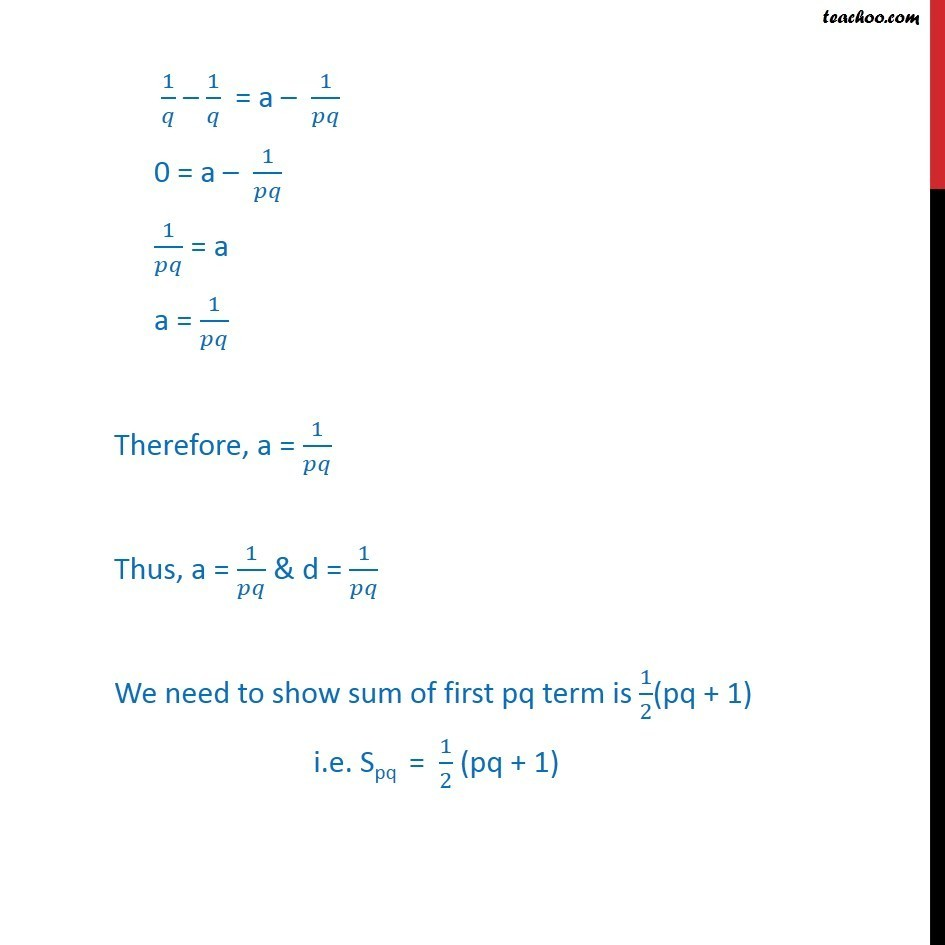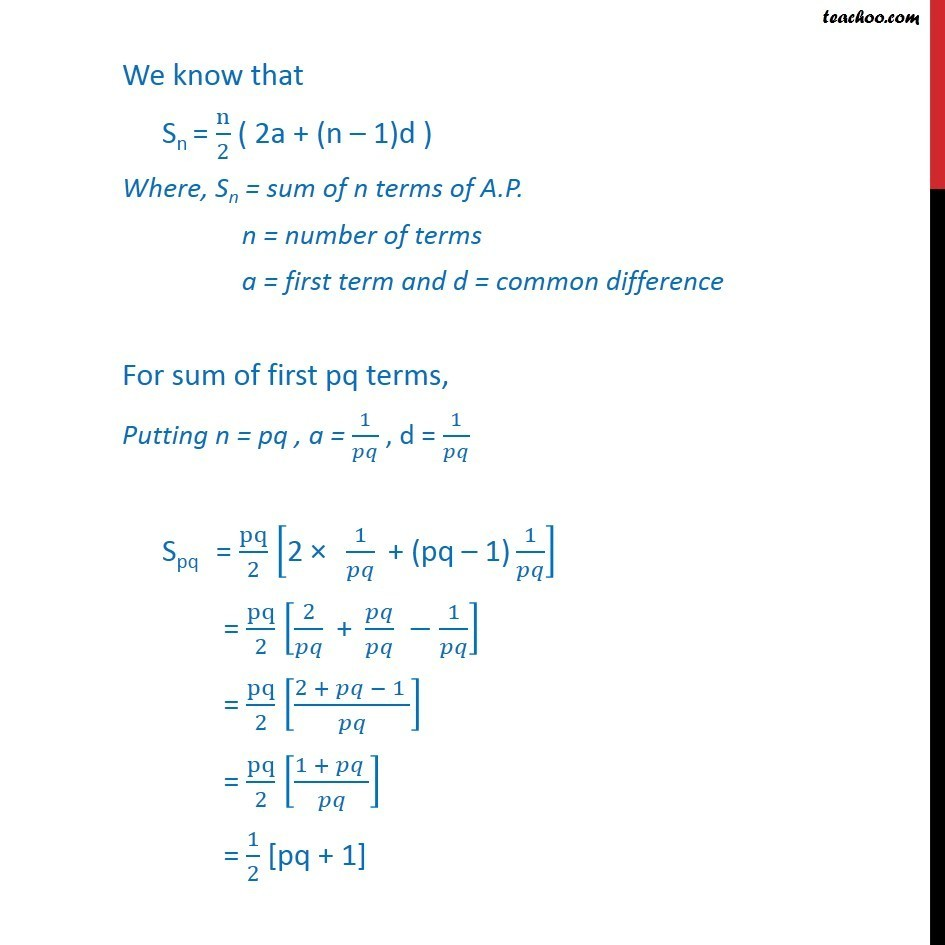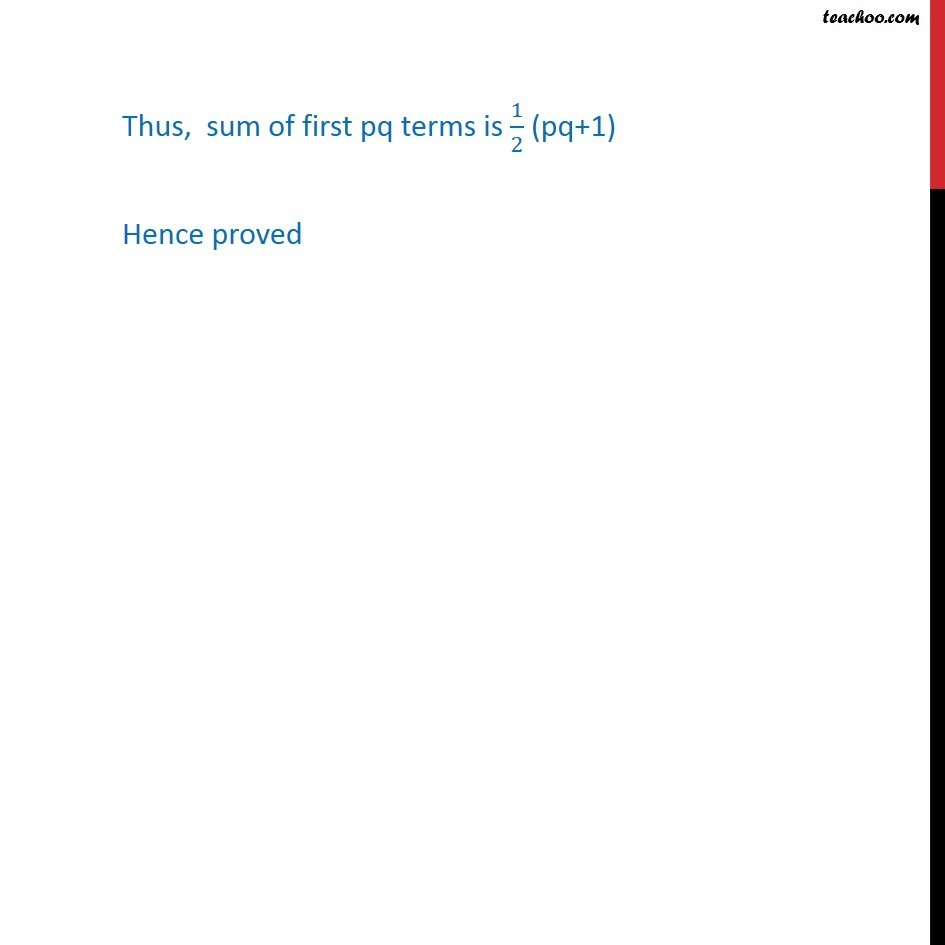Learn in your speed, with individual attention - Teachoo Maths 1-on-1 Class

### Transcript

Question5 In an A.P., if pth term is 1/q and qth term is 1/p , prove that the sum of first pq terms is 1/2 (pq + 1) where p q. We know that an = a + (n 1)d Where an is nth term of AP, n is the number of terms, a be the first term & d be the common difference of the A.P. It is given that pth term is 1/ i.e. ap = 1/q a + (p 1)d = 1/q Also, qth term of A.P = 1/p i.e. aq = 1/p i.e. a + (q 1)d = 1/p Now subtracting (1) from (2) i.e. (1) (2) [a + (p 1)d] [a + (q 1)d] = 1/ 1/ a + pd d [a + qd d] = 1/ 1/ a + pd d a qd + d = 1/ 1/ a a d + d + pd qd = 1/ 1/ 0 + 0 + pd qd = 1/ 1/ d(p q) = 1/ 1/ d(p q) = ( )/ d = ( )/( ( )) d = 1/ Now finding first term i.e. a Putting d = 1/ in (1) 1/ = a + (p 1)d 1/ = a + (p 1)1/ 1/ = a + / 1/ 1/ = a + 1/ 1/ 1/ 1/ = a 1/ 0 = a 1/ 1/ = a a = 1/ Therefore, a = 1/ Thus, a = 1/ & d = 1/ We need to show sum of first pq term is 1/2(pq + 1) i.e. Spq = 1/2 (pq + 1) We know that Sn = n/2 ( 2a + (n 1)d ) Where, Sn = sum of n terms of A.P. n = number of terms a = first term and d = common difference For sum of first pq terms, Putting n = pq , a = 1/ , d = 1/ Spq = pq/2 ["2 " 1/ " + (pq 1)" 1/ ] = pq/2 [2/ " + " / 1/ ] = pq/2 [(2 + 1 )/ ] = pq/2 [(1 + )/ ] = 1/2 [pq + 1] Thus, sum of first pq terms is 1/2 (pq+1) Hence proved